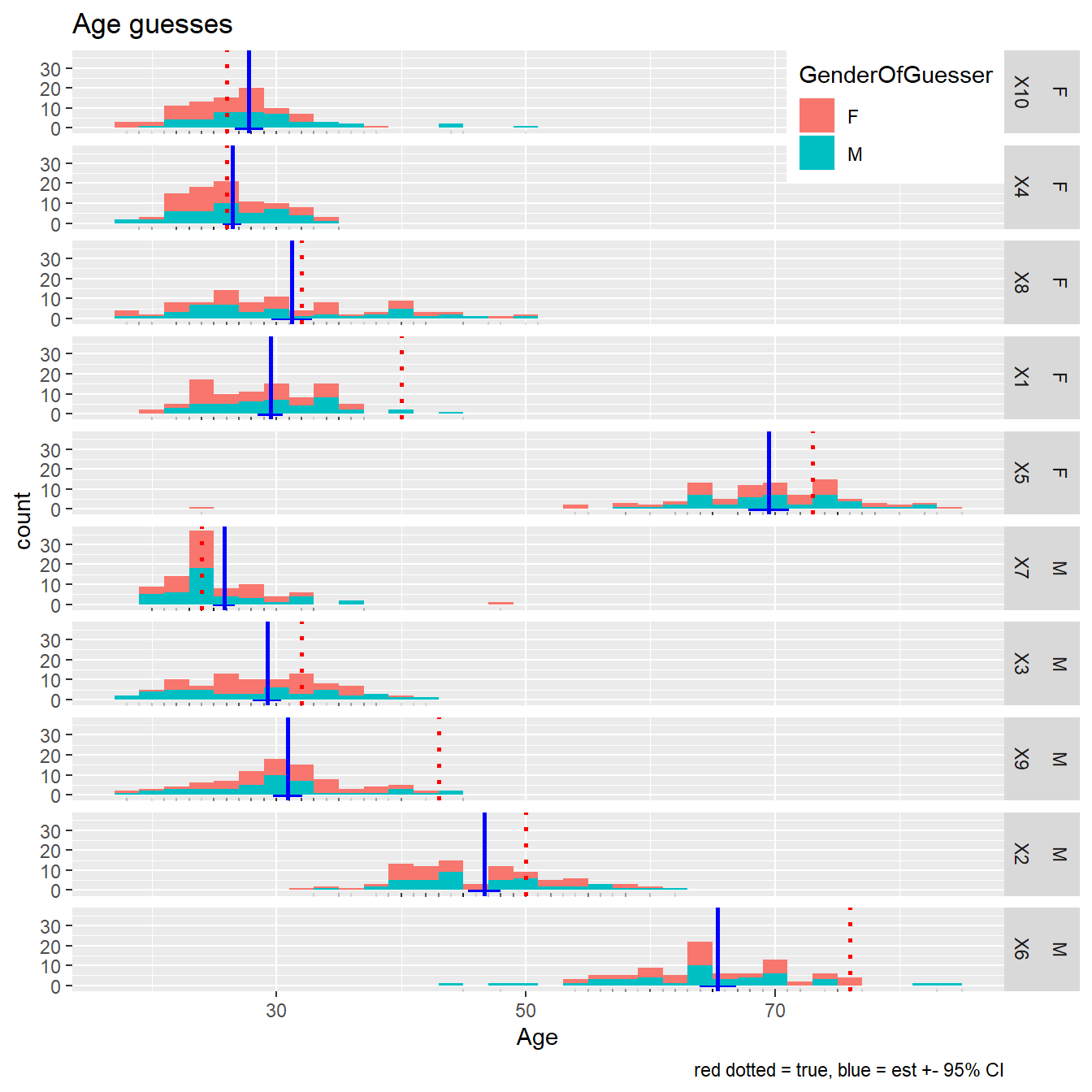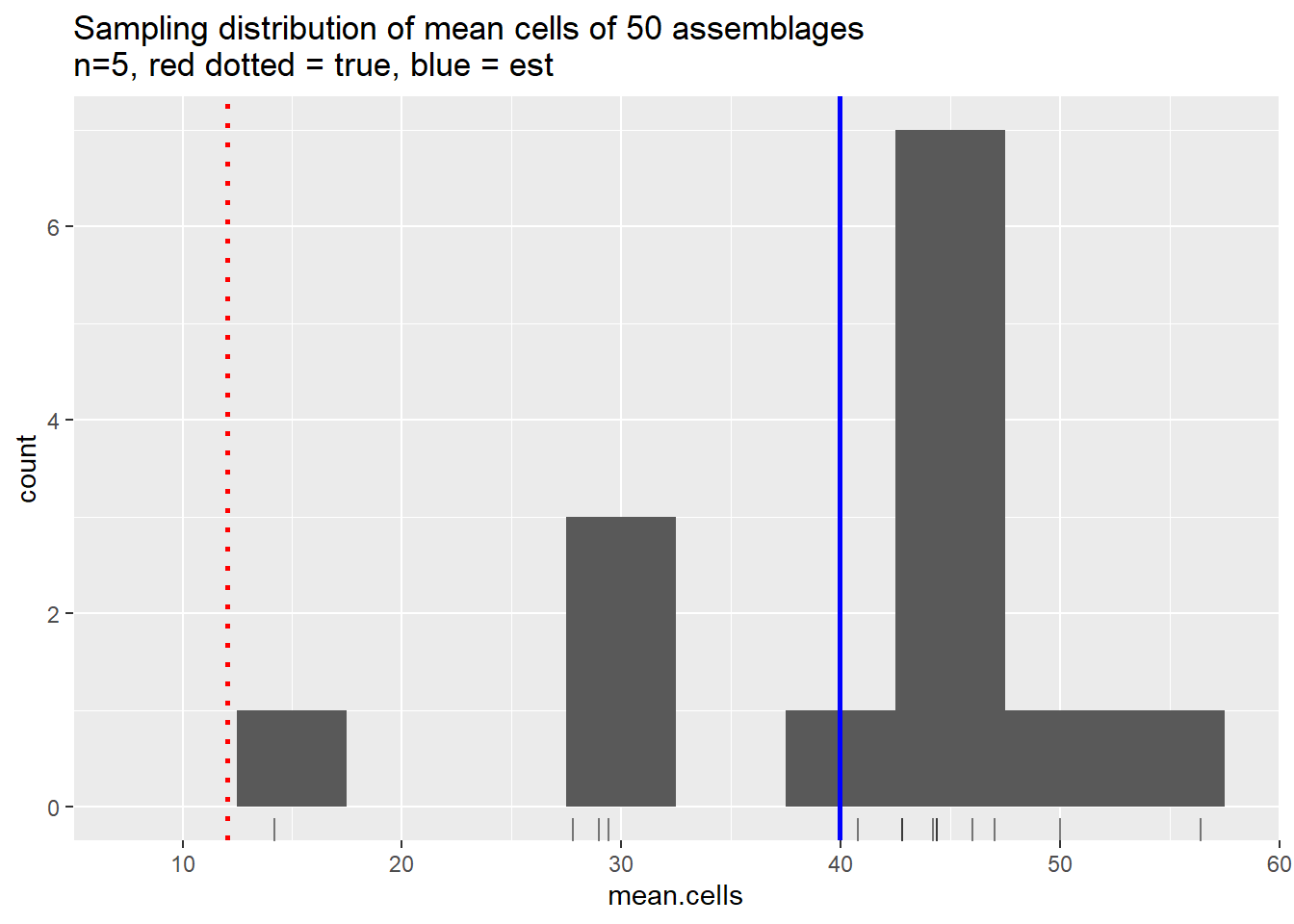Include your answers in this document in the sections below the rubric.

# Rubric

Answer the questions with the two data examples.

# Guess the ages example

## Read and reshape the data to produce summary table and plot

Erik has written a lot of code here to reshape, summarize, and combine the data in order to create the table and plot. You are encouraged to look at the code, run each line at a time and examine the results to understand the steps. The steps used are a common set of transformations for basic analysis.

library(tidyverse)
## -- Attaching packages ---------------------------------------- tidyverse 1.2.1 --
## v ggplot2 3.2.1     v purrr   0.3.2
## v tibble  2.1.3     v dplyr   0.8.3
## v tidyr   1.0.0     v stringr 1.4.0
## v readr   1.3.1     v forcats 0.4.0
## -- Conflicts ------------------------------------------- tidyverse_conflicts() --
## x dplyr::lag()    masks stats::lag()
# install.packages("gsheet")

library(gsheet)
# convert the spreadsheet to csv-formatted test
dat_ages_all <- gsheet2text(dat_ages_url)
## No encoding supplied: defaulting to UTF-8.
## Joining, by = "Image"
## Joining, by = c("Image", "Gender")

Below is a summary table for each image, and the associated plot. We will display the original images in class for comparisons.

# finally, display beautiful table
dat_ages_est_long
## # A tibble: 10 x 8
##    Image Gender TrueAge   Age    Bias SD_Age CI_lower CI_upper
##    <ord> <fct>    <dbl> <dbl>   <dbl>  <dbl>    <dbl>    <dbl>
##  1 X10   F           26  27.8   1.80    5.34     26.7     28.9
##  2 X4    F           26  26.5   0.451   3.66     25.7     27.2
##  3 X8    F           32  31.2  -0.791   7.80     29.6     32.8
##  4 X1    F           40  29.5 -10.5     4.86     28.5     30.5
##  5 X5    F           73  69.5  -3.52    7.86     67.8     71.1
##  6 X7    M           24  25.8   1.79    4.27     24.9     26.7
##  7 X3    M           32  29.3  -2.75    5.51     28.1     30.4
##  8 X9    M           43  30.9 -12.1     5.71     29.7     32.1
##  9 X2    M           50  46.7  -3.33    6.28     45.4     48.0
## 10 X6    M           76  65.4 -10.6     6.99     64.0     66.9
# plot data and create table
library(ggplot2)
p <- ggplot(dat_ages_long, aes(x = Age))
p <- p + geom_histogram(aes(fill = GenderOfGuesser), position="stack", binwidth = 2, alpha = 1)
p <- p + geom_rug(alpha = 1/8)
# true ages
p <- p + geom_vline(data = dat_ages_true_long, aes(xintercept = Age)
, colour = "red", linetype = "dotted", size = 1)
# est ages
p <- p + geom_vline(data = dat_ages_est_long, aes(xintercept = Age)
, colour = "blue", size = 1)
p <- p + geom_rect(data = dat_ages_est_long, aes(xmin = CI_lower, xmax = CI_upper, ymin = -1, ymax = 0)
, fill = "blue", alpha = 1)
p <- p + facet_grid(Gender + Image ~ ., space = "free")
# Legend: Put top-right corner of legend box in top -right corner of graph
p <- p + theme(legend.justification=c(1,1), legend.position=c(1,1))
p <- p + labs(title = "Age guesses", caption = "red dotted = true, blue = est +- 95% CI")
print(p)1. (1 p) From the table or plot, what is the overall pattern of age guesses based on the Gender and Age of the Image based on the Gender of the guesser?

2. (1 p) The sample who took the Guess the Ages survey were “Self-selected undergraduate and graduate students in Stat 427/527 this semester”. Define the population this sample was taken from.

3. (1 p) The population parameter being tested is “The mean age $$\mu_j$$ that people in the population would assess for each image $$j=1, \ldots, 10$$.” (Note, the population parameter is not the true age, rather, it is the mean assessed age. The bias difference of these two is an indicator of whether a person looks young or old for their age.) The sample statistic is $$\bar{Y}_j$$, the sample mean for each image. Give the name for and define the standard deviation for this sample statistic.

4. (2 p) Report and interpret the confidence interval for image X1.

# Lego example

## Draw samples, estimate the mean “cells” of 50 Lego assemblages

A “cell” is defined as a one-unit high square that includes a single lego circle. Some assemblages have a 1/5-high base; ignore this, it is only for structure.

Procedure:

1. Select a “representative or random sample” of 5 assemblages out of the bag.
2. Count the number of cells for each assemblage.
3. Calculate the sample mean of the 5 (to estimate population mean of the 50); you can use this R code by replacing the numbers: mean(c(1, 2, 3, 4, 5)).
4. Write your mean cell-count estimate on a portable white board in big numbers (3 decimal places) and lay it on your table (so other tables don’t see).
5. When all tables are done, hang up your board so everyone can see each table’s cell-count estimate.
6. Record all the estimates in the R code chunk below and plot the estimate of the sampling distribution of the mean cell count based on $$n=5$$.
# enter list of cells means here
#sam.lego <- data.frame(mean.cells = c(0, 0, 0, 0, 0, 0
#                                    , 0, 0, 0, 0, 0, 0))
sam.lego <-
data.frame(
mean.cells =
c(14.2, 29.4, 27.8, 40.8, 29.0
, 47.0, 42.8, 50.0, 44.2, 56.4
, 42.8, 44.4, 46.0, 44.4
)
)

# we'll fill this in after data is collected
#true.mean.cells = 0
true.mean.cells = 12.06

summary(sam.lego$mean.cells) ## Min. 1st Qu. Median Mean 3rd Qu. Max. ## 14.20 32.25 43.50 39.94 45.60 56.40 # plot data and create table library(ggplot2) p <- ggplot(sam.lego, aes(x = mean.cells)) p <- p + geom_histogram(binwidth = 5) p <- p + geom_rug(alpha = 1/2) # est mean cells p <- p + geom_vline(aes(xintercept = mean(sam.lego$mean.cells))
, colour = "blue", size = 1)
# true mean cells
p <- p + geom_vline(aes(xintercept = true.mean.cells)
, colour = "red", linetype = "dotted", size = 1)
p <- p + labs(title = "Sampling distribution of mean cells of 50 assemblages\nn=5, red dotted = true, blue = est")
print(p)1. (1 p) Adjust the histogram binwidth= to provide an informative representation of the distribution.